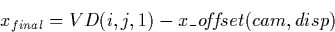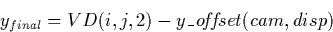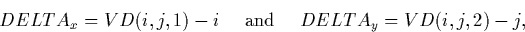Next: 12.6 Low-Dispersion Resampled Image Up: 12 Final Archive Data Previous: 12.4 Linearized Image FITS

12.5 Vector Displacement FITS File (VDLO/VDHI)

The VD defines the final SI coordinate values in the x (wavelength) and y (spatial) directions for every LI pixel. The final coordinates in SI space for any photometrically-corrected pixel in the LILO/LIHI are determined by:where i and j range from 1 to 768, and x_offset and y_offset are given in the following table.

 x_offset y_offset disp=L H L H LWP 100 297 LWR 100 250 SWP 130 490

The output displacements between the SI and LI coordinates are recoverable by:where i and j range from 1 to 768. xfinal and yfinal contain the final x and y coordinates in the SILO/SIHI. The x and y coordinates of the displacement vectors are stored as a 3-D primary array consisting of 768x768x2 elements. The displacements are coded as 32-bit, floating point numbers.

The XC allow the user to recover the calculated displacement vectors, mapping the science image (in raw space) to the ITF. For each of the approximately 500 (140 for low dispersion) points used to obtain the displacement between the science image and the corresponding level of the ITF, the binary table extension will contain the following columns of information: science image x-position (I*2), science image y-position (I*2), ITF x-position at position of best match (R*4), ITF y-position at position of best match (R*4), the cross-correlation coefficient (R*4), number of points used to calculate the coefficient (I*2), and the ITF level used in the correlation (I*2). The x and y positions correspond to the sample and line numbers in the RI. The resulting ITF positions of the best match are pre-filtered positions (before invalid matches have been identified and deleted) and will not necessarily correspond exactly to the photometric registration displacement components utilized to create the final displacement vector.

Basic keywords in the VDLO/VDHI headers and binary table extensions are shown in Table 12.7. Note that the CTYPE1 and CTYPE3 keyword values listed and as stored in the archived VDLO/VDHI are incorrect and should be interchanged. Unfortunately, this error was not discovered until the majority of images were processed and so was left uncorrected for consistency. Note also that the VDLO/VDHI will not be available for images processed at VILSPA, nor for images processed at GSFC after July 31, 1997.

Table 12.7:  VDLO/VDHI - Basic FITS Keywords
Keyword and value Description
 SIMPLE = T Standard FITS Format
 BITPIX = -32 IEEE single precision floating point
 NAXIS = 3 Three-dimensional image
 NAXIS1 = 768 Dimension along x-axis
 NAXIS2 = 768 Dimension along y-axis
 NAXIS3 = 2 Dimension along z-axis
 EXTEND = T Extensions are present
 CTYPE1 = ' '  Units x-axis
 CTYPE2 = 'PIXEL '  Units y-axis
 CTYPE3 = 'PIXEL '  Units z-axis
 BUNIT = 'PIXEL '  Pixel units
 TELESCOP= 'IUE '  International Ultraviolet Explorer
 FILENAME= 'AAAnnnnn.VDdd'  Filename(camera)(number).VD(disp)
 DATE = 'dd/mm/yy'  Date file was written
 ORIGIN = 'VILSPA '  Institution generating the file
 DATAMIN = nnnnn.n  Minimum pixel value
 DATAMAX = nnnnn.n  Maximum pixel value
 XTENSION= 'BINTABLE'  Table extension
 BITPIX = 8 Binary data
 NAXIS = 2 Two-dimensional table array
 NAXIS1 = 20 Width of table in bytes
 NAXIS2 = nnn Number of entries in table
 PCOUNT = 0 Number of bytes following data matrix
 GCOUNT = 1 Number of groups
 TFIELDS = 7 Number of fields in each row
 TFORM1 = '1I '  Count and data type for field 1
 TTYPE1 = 'XRAW '  Science image x-position
 TUNIT1 = 'PIXEL '  Unit is pixels
 TFORM2 = '1I '  Count and data type for field 2
 TTYPE2 = 'YRAW '  Science image y-position
 TUNIT2 = 'PIXEL '  Unit is pixel
 TFORM3 = '1E '  Count and data type for field 3
 TTYPE3 = 'XITF '  ITF x-position of best match
 TUNIT3 = 'PIXEL '  Unit is pixel
 TFORM4 = '1E '  Count and data type for field 4
 TTYPE4 = 'YITF '  ITF y-position of best match
 TUNIT4 = 'PIXEL '  Unit is pixel
 TFORM5 = '1E '  Count and data type for field 5
 TTYPE5 = 'XCOEFF '  Cross correlation coefficient
 TUNIT5 = ' '  Unitless
 TFORM6 = '1I '  Count and data type for field 6
 TTYPE6 = 'NPOINTS '  Number of points used
 TUNIT6 = ' '  Unitless
 TFORM7 = '1I '  Count and data type for field 7
 TTYPE7 = 'ITFLEVEL'  ITF level
 TUNIT7 = ' '  Unitless
 FILENAME= 'AAAnnnnn.XCdd'  Filename (camera)(number).XC(disp)
 EXTNAME = 'XCOEFF '  Cross correlation coefficientsNext: 12.6 Low-Dispersion Resampled Image Up: 12 Final Archive Data Previous: 12.4 Linearized Image FITS
Karen Levay
12/4/1997